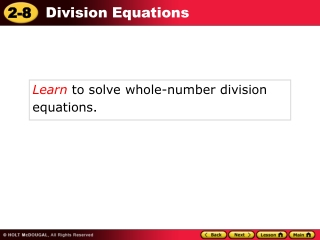DownloadDownload PresentationLearn to solve whole-number division equations .

# Learn to solve whole-number division equations .

Télécharger la présentation## Learn to solve whole-number division equations .

- - - - - - - - - - - - - - - - - - - - - - - - - - - E N D - - - - - - - - - - - - - - - - - - - - - - - - - - -
##### Presentation Transcript

1. Learn to solve whole-number division equations.

2. Recall that multiplication and division are inverse operations. If an equation contains division, solve it by multiplying on both sides to “undo” the division.

3. x = 5 7 x = 5 x is divided by 7. 7 x 7 = 7 5 7 x = 5 Check 7 35 ? = 5 7 ? 5 = 5 Additional Example 1A: Solving Division Equations Solve the equation. Check your answer. Multiply both sides by 7 to undo the division. x = 35 Substitute 35 forx in the equation. 35 is the solution. 

4. p 13 = 6 p 13 = p is divided by 6. 6 p 6 13 = 6 6 p 13 = Check 6 78 ? 13 = 6 ? 13 = 13 Additional Example 1B: Solving Division Equations Solve the equation. Check your answer. Multiply both sides by 6 to undo the division. 78 = p Substitute 78 forp in the equation. 78 is the solution. 

5. x = 9 2 x = 9 x is divided by 2. 2 x 2 = 2 9 2 x = 9 Check 2 18 ? = 9 2 ? 9 = 9 Check It Out: Example 1A Solve the equation. Check your answer. Multiply both sides by 2 to undo the division. x = 18 Substitute 18 forx in the equation. 18 is the solution. 

6. p 72 = 4 p 72 = p is divided by 4. 4 p 4 72 = 4 4 p 72 = Check 4 288 ? 72 = 4 ? 72 = 72 Check It Out: Example 1B Solve the equation. Check your answer. Multiply both sides by 4 to undo the division. 288 = p Substitute 288 forp in the equation. 288 is the solution. 

7. h Substitute 14 for height of aspen. h is divided by 3. 14 = 3 h 3 14 = 3 3 Additional Example 2: Application At Elk Meadows Park an aspen tree is one-third the height of a pine tree. height of pine height of aspen = 3 The aspen tree is 14 feet tall. How tall is the pine tree? Let h represent the height of the pine tree. Multiply both sides by 3 to undo the division. 42 = h The pine tree is 42 feet tall.

8. w Substitute 95 for Jamie’s weight. w is divided by 2. 95 = 2 w 2 2 95 = 2 Check It Out: Example 2 Jamie weighs one-half as much as her father. father’s weight Jamie’s weight = 2 Jamie weighs 95 pounds. How many pounds does her father weigh? Let w represent her father’s weight. Multiply both sides by 2 to undo the division. 190 = w Jamie’s father weighs 190 pounds.